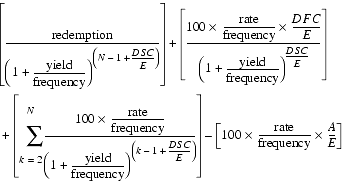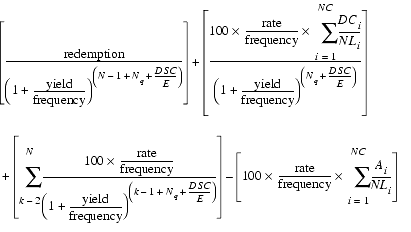ODDFPRICE

Description Computes the price of a security purchased during a first coupon period that is shorter or longer than the other coupon periods. In addition to the dates, you must specify the rate, redemption value, and yield expected.

Syntax ODDFPRICE ( settlement, maturity, issue, first_coup, rate, yield, redemption, frequency [, calendar_type])

Argument Description
settlement The date when the security is traded to the buyer. It must be before first_coup. Decimal values will be truncated to integers.
maturity The date the security expires and the remaining amount is paid to the investor. Decimal values will be truncated to integers.
issue The date the security becomes effective.
first_coup The date of the first interest payment.
rate The security's annual coupon rate.
yield The annual income produced by the security.
redemption The security's redemption value per \$100 face value. This is the amount paid at maturity that is not part of any final coupon payment.
frequency The number of interest payments per year. See The frequency Argument for more information.
[calendar_type] Optional. One of five methods of counting days for computing interest. See The calendar_type Argument for more information.

Remarks This function is useful in cases where the security's issuer does not pay interest for the first few months or years after issuing the security.

This function should be used only when the security's first coupon date is a different length than the other coupon dates AND the security was purchased during this odd coupon period.

If all coupon periods are of equal length, use PRICE. If the last coupon period is shorter or longer than the rest, use ODDLPRICE.

Equation when the first coupon period is shorter than the rest:...where the codes correspond to values computed using other functions, as shown in the following table.
Code Meaning Function
A Number of days from issue to settlement. COUPDAYBS
DSC Number of days from settlement to first_coup. COUPDAYSNC
DFC Number of days from issue to first_coup. COUPDAYSNC
E Number of days in a normal coupon period. COUPDAYS
N Number of coupons payable between settlement and maturity. COUPNUM

Equation when the first coupon period is longer than the rest:...where the codes correspond to values computed using other functions, as shown in the table below.

Note The software divides the odd first period into non-paying coupon periods in order to correctly calculate the price. These non-paying periods are referred to as pseudo-periods. The variable i represents the number of pseudo-periods. The first pseudo-period may be shorter than the rest of the pseudo-periods.
Code Meaning Function
Ai Number of days from issue to settlement. COUPDAYBS
DCi Number of days from issue to the first pseudo-period. If the first pseudo-period is the same length as the other pseudo-periods, this is the number of days in the pseudo-periods. COUPDAYSNC
DSC Number of days from settlement to first_coup. COUPDAYSNC
E Number of days in a normal coupon period. COUPDAYS
N Number of coupons payable between first_coup and maturity. (Fractional values are raised to integers.) COUPNUM
NC Number of pseudo-periods. (Fractional values are raised to integers.) COUPNUM
NLi Number of days in the pseudo-periods. COUPDAYS
Nq Number of pseudo-periods between settlement and first_coup. (Fractional values are truncated.) COUPNUM

Examples This function returns 104.2651:

ODDFPRICE("1/15/93","1/1/98","1/1/93","3/1/93",0.07,0.06,100,2)